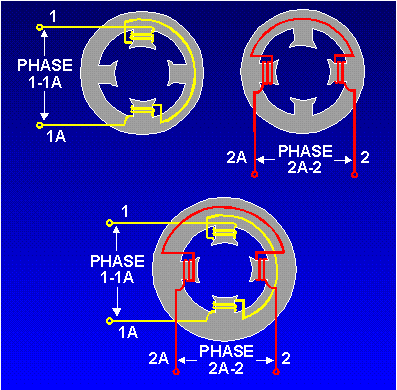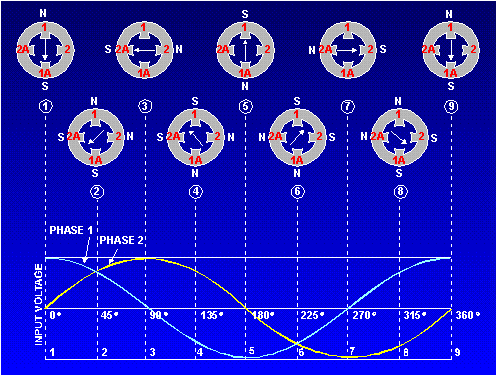rotating magnetic fields in their stators to cause their rotors to turn. The idea is simple. A magnetic field in a stator can be made to rotate electrically, around and around. ">Custom SearchROTATING MAGNETIC FIELDS The principle of rotating magnetic fields is the key to the operation of most ac motors. Both synchronous and induction types of motors rely on rotating magnetic fields in their stators to cause their rotors to turn. The idea is simple. A magnetic field in a stator can be made to rotate electrically, around and around. Another magnetic field in the rotor can be made to chase it by being attracted and repelled by the stator field. Because the rotor is free to turn, it follows the rotating magnetic field in the stator. Let's see how it is done. Rotating magnetic fields may be set up in two-phase or three-phase machines. To establish a rotating magnetic field in a motor stator, the number of pole pairs must be the same as (or a multiple of) the number of phases in the applied voltage. The poles must then be displaced from each other by an angle equal to the phase angle between the individual phases of the applied voltage. Q.4 What determines the number of field poles required to establish a rotating magnetic field in a multiphase motor stator?TWO-PHASE ROTATING MAGNETIC FIELD A rotating magnetic field is probably most easily seen in a two-phase stator. The stator of a two-phase induction motor is made up of two windings (or a multiple of two). They are placed at right angles to each other around the stator. The simplified drawing in figure 4-2 illustrates a two-phase stator. Figure 4-2. - Two-phase motor stator.If the voltages applied to phases 1-1A and 2-2A are 90 out of phase, the currents that flow in the phases are displaced from each other by 90. Since the magnetic fields generated in the coils are in phase with their respective currents, the magnetic fields are also 90 out of phase with each other. These two out-of-phase magnetic fields, whose coil axes are at right angles to each other, add together at every instant during their cycle. They produce a resultant field that rotates one revolution for each cycle of ac. To analyze the rotating magnetic field in a two-phase stator, refer to figure 4-3. The arrow represents the rotor. For each point set up on the voltage chart, consider that current flows in a direction that will cause the magnetic polarity indicated at each pole piece. Note that from one point to the next, the polarities are rotating from one pole to the next in a clockwise manner. One complete cycle of input voltage produces a 360-degree rotation of the pole polarities. Let's see how this result is obtained. Figure 4-3. - Two-phase rotating field.The waveforms in figure 4-3 are of the two input phases, displaced 90 because of the way they were generated in a two-phase alternator. The waveforms are numbered to match their associated phase. Although not shown in this figure, the windings for the poles 1-1A and 2-2A would be as shown in the previous figure. At position 1, the current flow and magnetic field in winding 1-1A is at maximum (because the phase voltage is maximum). The current flow and magnetic field in winding 2-2A is zero (because the phase voltage is zero). The resultant magnetic field is therefore in the direction of the 1-1A axis. At the 45-degree point (position 2), the resultant magnetic field lies midway between windings 1-1A and 2-2A. The coil currents and magnetic fields are equal in strength. At 90 (position 3), the magnetic field in winding 1-1A is zero. The magnetic field in winding 2-2A is at maximum. Now the resultant magnetic field lies along the axis of the 2-2A winding as shown. The resultant magnetic field has rotated clockwise through 90 to get from position 1 to position 3. When the two-phase voltages have completed one full cycle (position 9), the resultant magnetic field has rotated through 360. Thus, by placing two windings at right angles to each other and exciting these windings with voltages 90 out of phase, a rotating magnetic field results. Two-phase motors are rarely used except in special-purpose equipment. They are discussed here to aid in understanding rotating fields. You will, however, encounter many single-phase and three-phase motors. Q.5 What is the angular displacement between field poles in a two-phase motor stator?Integrated Publishing, Inc. - A (SDVOSB) Service Disabled Veteran Owned Small Business